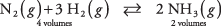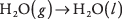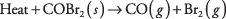## Le Chatelier's Principle

A valuable guide is available to assist you in estimating how chemical equilibrium will shift in response to changes in the conditions of the reaction, such as a modification of temperature or pressure. The French chemist Henri Le Chatelier realized in 1884 that if a chemical system at equilibrium is disturbed, the system would adjust itself to minimize the effect of the disturbance. This qualitative reasoning tool is cited as Le Chatelier's principle.

Begin by considering how an equilibrium system adjusts to a change in the concentration of any substance. At equilibrium, the concentrations of all substances are fixed, and their ratio yields the equilibrium constant. Le Chatelier's principle tells you that changing the concentration of a substance causes the system to adjust to minimize the change in that substance. The decomposition of carbonyl bromide provides an illustration:If the three gases in the reaction were at equilibrium and you then increased the carbon monoxide concentration, some Br 2 would combine with added CO to produce COBr 2 and thereby minimize the increase in CO. Alternatively, if you decrease the CO concentration, some COBr 2 would decompose to produce CO and Br 2 and thereby minimize any decrease in CO. Notice how the concentrations of all constituents shift to counteract the imposed change in a single substance. Of course, this shift does not affect the value of the equilibrium constant. Only a change in temperature can do that.

Notice how a change in pressure would affect the equilibrium reaction.which has an equilibrium constant at standard temperature and pressure that is calculated asAn increase in pressure will, according to Le Chatelier, cause the equilibrium to shift to minimize the pressure increase. Because the equilibrium reaction has more relative volumes on the left side, the pressure increase would be minimized by some N 2 and H 2 (total of four volumes) combining to form NH 3 (two volumes). Although the relative pressures of the gases have changed, the equilibrium constant still equals K. Conversely, a decrease in pressure would be minimized by the dissociation of some NH 3 (two volumes) to form N 2 and H 2 (four volumes).

The only reactions that are significantly affected by pressure are reactions involving gases in which the stoichiometric coefficients of the gases add to different values on the two sides of the reaction. Pressure, therefore, would not affect the equilibrium ofwhich has two volumes on each side. But pressure would affect the equilibrium forwhich has three volumes on the left and only two on the right. In this latter example, an increase of pressure induces the forward reaction, and a decrease in pressure causes the reverse reaction. Notice that the effect of varying pressure is to cause the concentrations of the various gases to shift, without any change in the equilibrium constant.

A change in temperature, however, does force a change in the equilibrium constant. Most chemical reactions exchange heat with the surroundings. A reaction that gives off heat is classified as exothermic, whereas a reaction that requires the input of heat is said to be endothermic. (See Table 1.) A simple example of an endothermic reaction is the vaporization of water:which absorbs 40.7 kilojoules per mole. The converse condensation reactionis exothermic because it releases 40.7 kilojoules per mole. A change of state is not required for heat to be involved in a reaction. The combustion of methaneinvolves only gases, yet this endothermic reaction absorbs heat.In a system at chemical equilibrium, there are always two opposing reactions, one endothermic and the other exothermic.

You can now consider how a change in temperature affects chemical equilibrium. In accordance with Le Chatelier's principle, the equilibrium constant changes to minimize the change in temperature. For endothermic reactions, an increase in temperature can be minimized by utilizing some of the heat to convert reactants to products, shifting the equilibrium to the right side of the reaction and increasing the value of K. For exothermic reactions, an increase in temperature can be minimized by using some of the heat to convert “products” into “reactants” and shifting the equilibrium toward the left side, reducing the value of K.

For a chemical system at equilibrium, an increase in temperature favors the endothermic reaction, whereas a decrease in temperature favors the exothermic reaction. The equilibrium reaction written asis endothermic when proceeding to the right and exothermic when proceeding to the left. Its equilibrium constant, given bymust increase if the temperature increases. Conversely, a lowering of the temperature will cause K to decrease.

Please realize that the effect of temperature on the equilibrium constant depends on which of the two opposing reactions is exothermic and on which is endothermic. You must have information on the heat of a reaction before you can apply Le Chatelier's principle to judge how temperature alters the equilibrium.

The next two practice problems refer to the following reaction, which is endothermic in the forward direction.• How would an increase in total confining pressure affect the masses of the two nitrogen oxides?
• How would an increase in temperature affect the masses of the two nitrogen oxides?